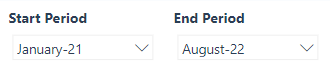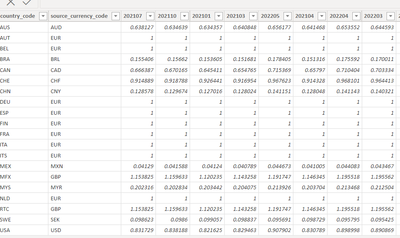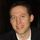cancel
Showing results for
Did you mean:Helper III

## select column dynamically in dax measure - is it possible?

Hi,

In my report I have a separate start and end filter for selecting time period.I have to calculate sellout amount to EUR, by using a sellout column in sales table and the exchange rate from the exchange rate table.

The tricky part is, that if Aug 22 is selected, they want everything to be calculated with Aug 22 ex rate. If Jul 22 is selected as end period, they want everything to be calculated on that exchange rate.

First I had an ex rate table like this:and it was connected with many to many relationship to the sales table by the country code column.

Sales amount was calculated with the help of a lookup function. The disadvantage of the Lookup function is the performance.  RELATED function would be much faster, but for that I would need a one to many relationship.

Now, what I am trying to do, is that I modified the exchange rate table, pivoted it, so that I have the ex rates in different columns and I can now establish the one to many relationship to the sales table.I can use now the RELATED function, but as each month is in a different column, I would have to add each and every column in the code, in the switch function. (The code always have to use the exchange rate of the selected end period)

Is it possible to reference the column name with a measure, instead of adding 50-60 lines of code,  each month one by one? It should always use the column name that is identical with the selected end period.

Thank you!

`Sellout Amount EUR test = VAR ex_ratedate = SELECTEDVALUE(Endperiod[yearmonth number])RETURNSUMX(sales, sales[sellout]*SWITCH(TRUE(), ex_ratedate=202101, RELATED(exchange_rate),ex_ratedate=202102, RELATED(exchange_rate), RELATED(exchange_rate)))`Super User

See if you can adapt the solution below. It uses a bridge table to solve the many-to-many relationship. In your model, CountryCode will be the bridge table. This approach allows you to avoid pivoting the exchange rate table.

Proud to be a Super User!